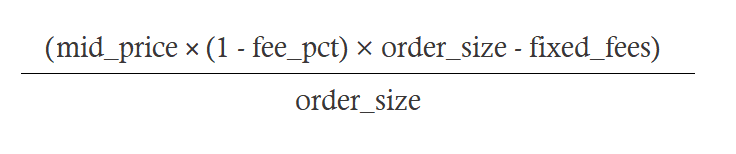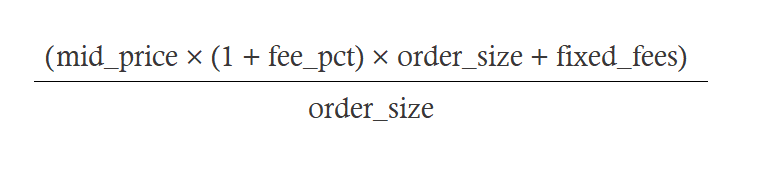Updated as of v0.28.0

This feature lets you take into account the transaction cost incurred on the exchange from bid and ask orders. For a bid order, it reduces the bid order price by the fee. For the ask order, it reduces the price by the fee. This allows you to consider the transaction cost to profit (or not incur a loss).

Note

This puts your order prices further away from the mid-price.

## add_transaction_costs¶

Whether to enable adding transaction costs to order price calculation.

Prompt:

Do you want to add transaction costs automatically to order prices? (Yes/No)
>>> Yes


## How it works¶

When the add_transaction_costs parameter is set to True, the client adds the transaction costs to the prices and adjusts the price proposal.

Type config add_transaction_costs to set the value for the parameter. If you respond Yes, the parameter is set to True, and if you type No, the parameter is set to False. This parameter is set to False by default.

Note that we currently display warnings if the adjusted price post adding the transaction costs is 10% away from the original price. If the buy price with the transaction cost is zero or negative, it is not profitable to place orders, and orders will not be placed.

## Order price calculation with transaction cost¶

Below, fee_pct refers to the percentage maker fees per order (generally common in Centralized exchanges), while fixed_fees refers to the flat fees (generally common in Decentralized exchanges).

### Calculating the bid order price¶### Calculating the ask order price¶### Example - when transaction cost is important¶

You are market making for the ETH-USD pair. The order_amount parameter is set to 1, and the bid_spread and ask_spread as set to 1 (representing 1%). Suppose the mid-market price of ethereum and USD is $200. When the add_transaction_costs is set to False, the bid order price is 1% below the mid-market price, and the ask order price is 1% above the mid-market price,$198 and $202, respectively. Suppose the fee percentage (fee_pct) is 1% and the fixed fee (fixed_fees) is$0.50 for each transaction. The spread above does not take into account these transaction costs and could hurt your profit. Your potential profit without taking into account the fees is $4. The fees incurred could be 1 dollar plus 1% times the value traded 1% x (199 + 200), which equals$3.99. Instead of profiting $4, you are losing$4 - ( $3.99 +$1) = - $0.99. When the add_transaction_costs is set to True, (using the formula above), the ask order price is$203, and the bid order price is $197. The profit before the transaction fees is$6. The transaction fees amount to $1 + .01 ($197 + $203) =$5.

Thus, your profit is \$1. Here, adding the transaction costs to the calculations was the difference between a loss and a profit.